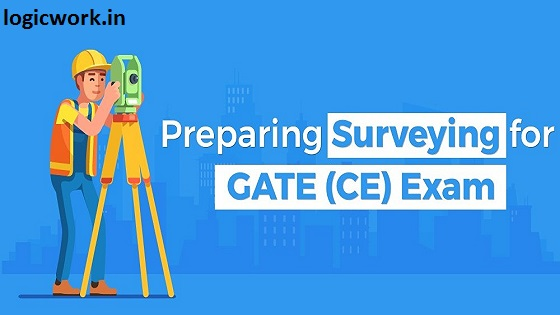# How to Prepare Geomatics/Surveying Engineering for GATE Civil?

In this blog, I will be discussing a small and scoring subject of Civil Engineering: Surveying and help you in preparing for Geomatics/Surveying Engineering. It is easy, takes less time, and is mostly based on geometry and trigonometry. Even one round of thorough study will be enough for this subject.

An analysis of the last 5 years of GATE papers reflects that Surveying carries 3-5 marks.

#### The time required for preparation Geomatics/Surveying Engineering

12-15 days (if you have 8-10 month of preparation) with 4 hours daily
7 days (if you have 4-5 months of preparation) with 7-8 hours daily

Let’s start our topic-wise discussion. It is advisable to prepare separate notes for formulae and for working out numerical questions. Approximate time required for each chapter is mentioned along with it which can be varied according to individual interests. The textbook(s) to be referred are mentioned in a separate comprehensive blog which you should refer.

###### 1. BASIC CONCEPTS (1 DAY)

Principles of surveying, the scale of a map (RF), measuring scales (plane scale, diagonal scale, Vernier scale, microscale, chord scale), errors due to shrinkage of map & wrong measuring scale, accuracy & precision. Practice 3 problems each on Vernier scale & errors in the map.

###### 2. LINEAR MEASUREMENTS & CHAIN SURVEY (1 DAY)

This is a very basic chapter and you can read through most of the parts. Chain survey instruments (different types of chains, tape, staff, peg, etc), ranging out a survey line, error due to incorrect chain, chaining on sloping ground (hypotenusal allowance), tape corrections (temperature, slope, standardization, misalignment, pull, sag, normal tension, reduction to MSL), limiting length of offset, obstacles in chain surveying. Prepare formula sheet & practice numerical of errors & corrections.

###### 3. ANGULAR MEASUREMENTS & COMPASS SURVEY (2-3 DAYS)

This is an important topic & needs practicing. Systems of bearing (WCB/RCB & conversions), Forbearing-Backbearing, included angles, types of the compass (prismatic & surveyor, comparison), magnetic declination (true bearing & magnetic bearing), dip, local attraction & correction of bearings.

And just completing these topics or chapter isn’t enough. As soon as you complete the part of the subjects start taking online GATE test series for CE.

###### 4. PLANE TABLE SURVEY (1 DAY)

This is a very small theoretical topic. Read through terms like resection, radiation, intersection, Lehman’s rule.

###### 5. Leveling (3 DAYS)

This is the most important topic in Surveying. Both Heights of instrument method & Rise/Fall method are important; practice numerical. Inverted staff reading, curvature & refraction corrections, distance to visible horizon (lighthouse-ship questions) are also important. Also, practice questions on reciprocal leveling and sensitivity of level tube. Trigonometrical leveling is also a part of this topic which can be just solved by basic trigonometry principles.

###### 6. TRAVERSE SURVEYING & OMITTED MEASUREMENTS (1-2 DAYS)

Latitude and Departure, dependent and independent co-ordinates, inversing, closing error, balancing the traverse (Bowditch method, transit rule & axis method), omitted measurements (completing the table).

###### 7. CONTOURING (1/2 DAY)

A very small topic, just read once. Contour interval, contour gradient, horizontal equivalent, characteristics of contours (and exceptional cases like a vertical hill or tilted hill where contours cross) are some terms you should be familiar with.

###### 8. TACHEOMETRY (1 DAY)

Stadia hair method, distance formula (D=kS+c: different cases like horizontal Line of sight (LOS), inclined LOS w/t vertical staff, inclined LOS w/t staff normal), tangential method (direct trigonometry). Most of the questions are trigonometry based, you should just know about distance formula & terms in that formula (k, c, f, d, D, S)

###### 9. HORIZONTAL & VERTICAL CURVES (1 DAY)

Relation b/w radius & degree of curve, various chainage lengths in a simple curve, linear & angular methods of setting a simple curve (just read), vertical curves (summit & valley), rate of change of gradient, length of vertical curve (in terms of no. of chain lengths) & its parabolic variation, transition curves.

*NOTE: This topic can be either studied in Surveying or Transportation (Highways).

###### 10. ERRORS & ADJUSTMENTS (1 DAY)

Significant digits, error in addition/subtraction/multiplication/division of quantities, the weight of observations & law of weight, standard deviation & variance, probable errors (with or without the weight of single measurement, average, the sum of measurements). You should practice 2-3 numerical of each topic & write the formulas in a formula sheet.

###### 11. PHOTOGRAMMETRY (1 DAY)

Focal length, the scale of a photograph, vertical photo & overlapping of vertical photos (calculation of a number of photos required). This is a small topic but only recently introduced; chances are that question might be asked from this topic. Practice 2 numerals each.

###### 12. and 13. REMOTE SENSING & GPS (–)

You cannot actually prepare much for these topics. These are general awareness based. You can go through previous questions, test-series questions, and Wikipedia knowing something related to these topics.